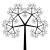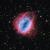# Hexahedron## Hexahedron Cube

I created a hexahedron (cube), which is a polyhedron platonic shape.# Introduction

Platonic solids are polyhedron which satisfy 3 conditions

1. all its faces are congruent convex regular polygons,
2. none of its faces intersect except at their edges, and
3. the same number of faces meet at each of its vertices

## Hexahedron

The most commonly recognised platonic solid, also known as a cube.